# The AVL algorithm for balancing a Binary Search Tree.

## By Nicholas Duchon, Oct 14, 2011

### Definitions

Much of this presentation follows the presentation of AVL trees in Data Structures and Algorithms in Java, 3rd edition, pp 421-431, ISBN 0-471-46983-1, 2004.

Let us assume that the BST is defined with:
• left < node < right
We will not allow repeats in these trees.

The algorithm uses a balance factor at each node based on the height of that node. So we need to define these terms.
• Height:
• The height of a node is one more than the length of the longest path from the node to a leaf of the subtree with the node as the root.
• Thus, the height of a leaf is 1.
• The height of null children is 0.
• Balance Factor:
• The balance factor of a node in a binary tree is the absolute value of the height of the left child minus the height of the right child.
• AVL Balanced:
• A binary search tree is AVL balanced if the balance factor at every node is no more than 1, meaning either 0 or 1.

### Test Cases

Notation:
• U = unbalanced node
• C = child of unbalanced node
• G = grandchild of unbalanced node
• N = target value that was just inserted
 Comments Numbers Changes in Height from Initial BST Initial BST Balanced BST - one rotation Second rotation Left Rotation Unbalanced at 1 N > U N > C left - right = -2 1 2 3 U = U - 2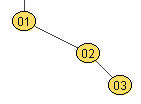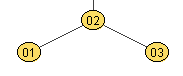Not needed Right Rotation Unbalanced at 3 N < U N < C left - right = 2 3 2 1 U = U - 2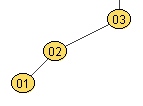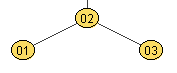Not needed Right-Left Rotation Unbalanced at 2 N > U N < C left - right = -2 2 1 5 4 6 3 U = U - 2 G = G + 1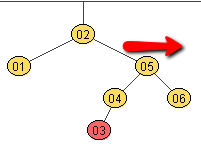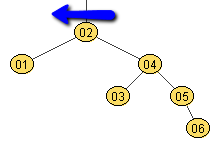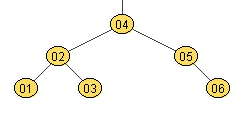Left-Right Rotation Unbalanced at 5 N < U N > C left - right = 2 5 2 6 1 4 3 U = U - 2 G = G + 1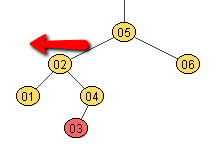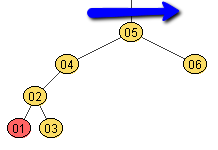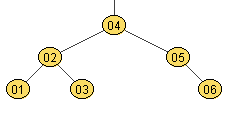For example, I have labelled the following tree with values and the heights of the nodes in parentheses.
You can generate this tree adding nodes in the level order, the first is the original tree, and the second adds the unbalanced node 87.
I have used space delimiters since that is the default for Scanner, and using Scanner.nextInt becomes simple.
• 44 24 64 16 32 51 88 14 17 28 42 50 54 81 94 12 48 62 78 85 92 96 73 83 86 95
• 44 24 64 16 32 51 88 14 17 28 42 50 54 81 94 12 48 62 78 85 92 96 73 83 86 95 87

 44 24 64 16 32 51 88 14 17 28 42 50 54 81 94 12 48 62 78 85 92 96 73 83 86 95 All the nodes in the tree above are balanced.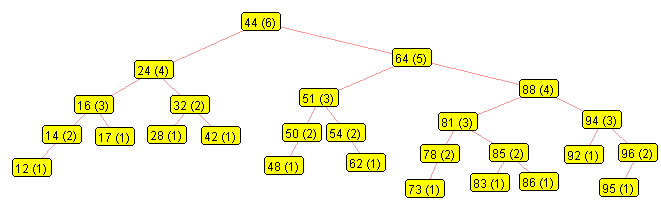We will now add 87 to the tree, and from that node up, we recompute the heights and balance factors. In this case, we see that node 64 is not balanced since the heights of its children are 3 and 5 respectively. Here, we have labelled those nodes: N (87) - the node just inserted U (64) - the node where the unbalance was detected C (88) - that node's child, which we tracked during the upward path from the inserted node (87) G (81) - the unbalanced node's grandchild, also tracked during the upward path from (87) P (44) - the parent of the unbalanced node. In this case, we have : N > U and N < C So we should do a Right-Left rotation: Right on C (88), then Left on U (64)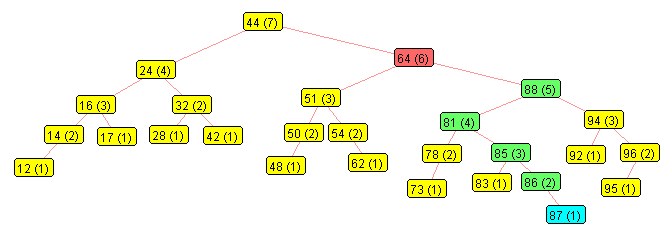Right on C (88): U right new= C left old (81) C left new = G right old (85) G right new = C (88)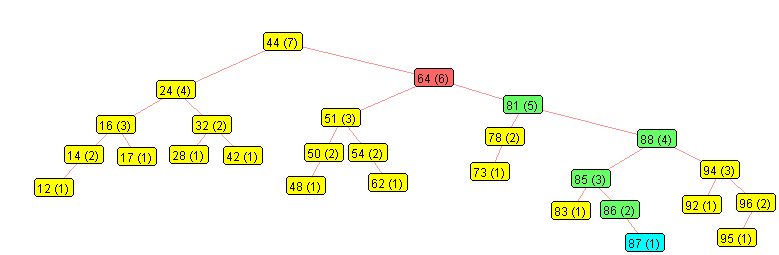Now left on U (64): P right new = G = U right current (81) U right new = G left = U right left current (78) G left = U (64)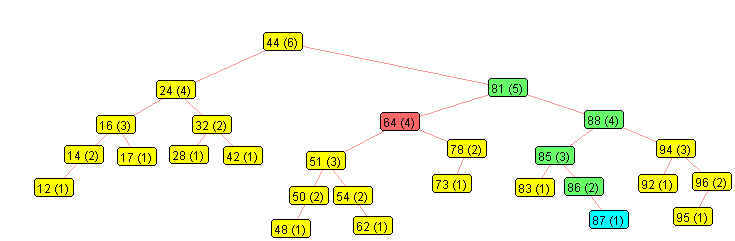### Algorithm:

• U = unbalanced node
• C = child of unbalanced node
• G = grandchild of unbalanced node
• N = target value that was just inserted
• T = pointer to new root of balanced subtree
parent of U or pointer to root of tree if U is the root
T = U
U.height = U.height - 2
if N > U
if N < U.right
U.right = Right Rotation on U.right (U.right.left++)
T = Left Rotation on U
else
if N > U.left
U.left = Left Rotation on U.left (U.left.right++)
T = Right Rotation on U
return T